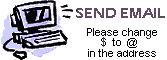Columnar Transposition Ciphers

A columnar transposition cipher is a simple hobbyist cipher in which the order of the letters in a message are changed, but the letters themselves are left unchanged.
The cipher is performed by writing the message into a grid, and then taking the letters out in a different order. The letters are written into the grid straight across the rows. Here we have written THIS IS A SAMPLE MESSAGE into a 5-column grid.

 T H I S I S A S A M P L E M E S S A G E

The message is taken out of the grid reading down the columns. In the simplest case, we would simply go straight down the columns from left to right. In this case the result is TSPS HALS ISEA SAMG IMEE. By convention, the letters are grouped by fives for transmission, so the final cipher would be

TSPSH ALSIS EASAM GIMEE

This would be trivial to solve. The only thing the opponent would need to do is guess the number of columns, or just start with 2 columns and work up. To make a more challenging cryptogram the order of reading out the columns is changed.
Instead of a fixed order, a key is used to determine the order. The key is a rearrangement of the column numbers. For example, with 5 columns, one possible key is 1,4,2,5,3. A different key is used for each message. The key is written at the top of the grid, so that each column is marked with one number from the key. When the text is read out, the columns are taken in the order prescribed by their marks.
Using the sample message above, and the key 1,4,2,5,3 we would have the grid

 1 4 2 5 3 T H I S I S A S A M P L E M E S S A G E

The column marked 1 is read out first, TSPS, then the column marked 2, ISEA, and so forth. This process gives TSPS ISEA IMEE HALS SAMG. As usual, the letters are regrouped into fives, thus

TSPSI SEAIM EEHAL SSAMG

When the number of letters in the cryptogram is an exact multiple of the number of columns (that is, when every column has the same number of letters) the method is called complete columnar transposition. If the length of the message may not be an exact multiple, the method is called incomplete columnar transposition.

To solve a columnar transposition, you first guess the number of columns (or, you try the following procedure for each grid width you think is likely). Then you determine the number of rows by dividing the length of the message by your guess at the number of columns. For example, suppose you suspect there are 8 columns, and the message length is 50. You divide 50 by 8 to get 6, with a remainder of 2. So there would be 6 full rows plus an extra row with only 2 letters.
Next you determine where in the message each column begins and ends. In the case above, the first column (that is, the first one that was read out of the grid) begins with the first letter of the message, and ends with either the 6th or 7th letter. The second column must begin with either the 7th or 8th letter, and end with either the 16th, 17th or 18th letter.
One popular method is to write each of these columns onto a vertical strip of cardboard, and then play with the order of the strips. At first, you look for likely letter pairs. For example, an H is often preceded by T, S or C. You try to find pairs of strips where most of the letters are followed by letters that usually follow them in English. For example, E is most commonly followed by A, N, R or S.
After you have found a likely pairing, try to extend it by placing a third strip to the left or right of them. Now short words and common fragments of words, like THE, AND and INT should begin to appear. From this point on you start to build words until the complete order of the strips is determined.Send us an email to submit your answers, to ask for help, or to submit new cryptograms. This site grows when you contribute puzzles. Be sure to change the \$ to an @ in our email address.